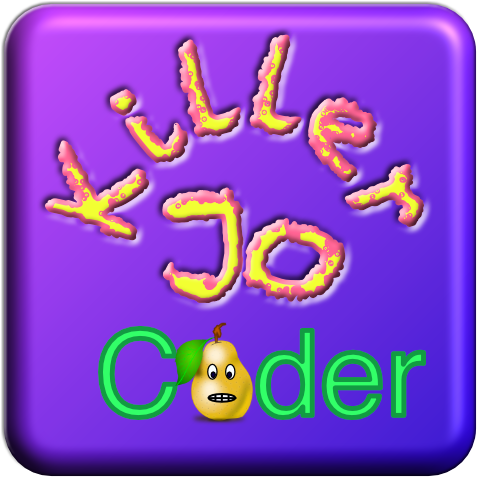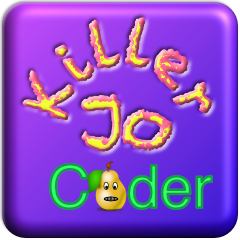# I NEED HELP (pls)

@Bri_G @dave1707 have a question for a game: how can you execute a command after a certain time? I would like to make it into an if (which is in a function draw) that after 5 seconds the command should be executed: background(255) is that possible I have been trying this for almost 1 hour

@killer.Jo - post us some of your code and we’ll correct what’s needed.

Here’s something I threw together.

``````
viewer.mode=FULLSCREEN

function setup()
rectMode(CENTER)
b1=color(255)
b2=color(255)
end

function draw()
background(255, 169, 0)

fill(b1)
rect(300,300,140,50)
fill(0)
text("    press then\nwait 5 seconds",300,300)

fill(b2)
rect(300,500,140,50)
fill(0)
text("    press then\nwait 10 seconds",300,500)

if s5 then
sprite(asset.builtin.Planet_Cute.Character_Horn_Girl, 450, 300)
end
if s10 then
sprite(asset.builtin.Planet_Cute.Character_Cat_Girl, 450, 500)
end
end

function x5()
s5=true
end

function x10()
s10=true
end

function touched(t)
if t.x>300-70 and t.x<300+70 and t.y>300-25 and t.y<300+25 then
b1=color(0, 218, 255)
tween.delay(5,x5)
end
if t.x>300-70 and t.x<300+70 and t.y>500-25 and t.y<500+25 then
b2=color(255, 0, 172)
tween.delay(10,x10)
end
end

``````

@Killer.Jo - here’s another way to do it.

``````
-- killerJo timer

function setup()
--
sW, sH, cW, cH = WIDTH, HEIGHT, WIDTH//2, HEIGHT//2
-- initialize timer
startTime = os.time()
end

function draw()
--
background(40, 40, 50)
elapsedTime = os.time() - startTime
timer = "Current elapsed time is : "
output.clear()
print(elapsedTime)
showSeconds()
triggerSprites()
end

function showSeconds()
--
fill(255)
font("AmericanTypewriter-Bold")
fontSize(32)
text(timer..elapsedTime,cW,900)
end

function triggerSprites()
--
if elapsedTime > 4 then
sprite(asset.builtin.Tyrian_Remastered.Boss_C,cW,756)
end
if elapsedTime > 9 then
sprite(asset.builtin.Tyrian_Remastered.Boss_D, cW, 256)
end
end

``````
``````
-- Killer Jo
viewer.mode = FULLSCREEN
viewer.retainedBacking = true

-- Definiere und initialisiere die Variablen
local GameStarts = false
local Start = false
local box01 = false
local Level02WIN = false
local level03 = false
local Apfelklik = false
local elapsedTime = 0
local clicks = 0
function setup()
Background = color(37)
LVL2txt = "Es ist nicht 2"
Island = "Deine Insel"
music(asset.documents["Lange Straße 20.m4a"])
end

function draw()
if not GameStarts then

background(Background)
sprite(Startbutton, 550, 500, 100, 100)
sprite(mainMenButton, 200, 750, 70, 70)
fill(255, 28, 0)
font("ArialRoundedMTBold")
sprite(Startbutton, 550, 300)
end

if CurrentTouch.state == BEGAN then
if CurrentTouch.x >= 200-75 and CurrentTouch.x <= 200+75 and
CurrentTouch.y >= 750-19 and CurrentTouch.y <= 750+19 then
end
end

end

if CurrentTouch.state == BEGAN then
if CurrentTouch.x >= 500-75 and CurrentTouch.x <= 600+75 and
CurrentTouch.y >= 500-19 and CurrentTouch.y <= 500+19 then
Start=true

end
end

if Start then
background(255)
sprite(box, 800, 750)
sprite(box, 250 , 500)
sprite(box, 800, 150)

if CurrentTouch.state == BEGAN then
if CurrentTouch.x >= 800-75 and CurrentTouch.x <= 800+75 and
CurrentTouch.y >= 150-19 and CurrentTouch.y <= 150+19 then
box01=true

end
end

if box01 then
background(255)
fontSize(25)
text(LVL2txt, 550, 700)
sprite(box, 550, 500)
sprite(box, 550, 300)
sprite(box, 500, 150)
fill(255, 237, 0)
font("ArialRoundedMTBold")
text("1", 550, 500)
text("2", 550, 300)
text("3", 500, 150)
if CurrentTouch.state == BEGAN then
if CurrentTouch.x >= 550-75 and CurrentTouch.x <= 550+75 and
CurrentTouch.y >= 500-19 and CurrentTouch.y <= 500+19 then
Level02WIN=true
end
end
if Level02WIN then

-- Verzögerte Animation starten
if CurrentTouch.state== ENDED then
HEY=true
end

if HEY then
-- das war tag 01
-- Jetzt kommt Tag 02!

background(0, 255, 216)

fill(255, 0, 225)
font("ArialRoundedMTBold")
fontSize(50)
text("Deine Insel", 550, 750)
-- Hier die Buttons
sprite(asset.documents.Start_buttonKJ, 850, 150, 110, 110)
sprite(asset.documents.Skineditor, 850, 350, 110, 110)
sprite(asset.documents.Islamd, 550, 700, 110, 110)

-- Hier die Texte
fontSize(25)
text("Start", 850, 150)
text("Editor", 850, 350)
text("Island", 550, 700)
fontSize(10)
text("Hier gibt's nicht viel zu sehen...", 150, 500)

if CurrentTouch.state == ENDED then
if CurrentTouch.x >= 550-75 and CurrentTouch.x <= 550+75 and
CurrentTouch.y >= 700-19 and CurrentTouch.y <= 700+19 then
Farm=true
end
if Farm then
background(25)
text("Das ist deine Farm!", 550, 750)
end
end
end
end
end
end

end

``````

I have created a big code that I plan to develop a game that never gets boring. Because I’m often bored too. That’s why I’m playing the game. But in this code there is an error, although when you are in your farm and you type (press) you come back to the main menu. I don’t want that. I don’t really have a real idea what to do yet because I don’t just want to do level. Maybe you could give me ideas would be cool
Edit: Unfortunately, you can’t see my dropbox stuff. But can you do that somehow?
Edit2: 4 mega bytes are a bit small. Nevertheless, the other question was how can I fix the error?@Killer.Jo If you zip your code and post the zip file, that includes your document sprites.

@dave1707 4 mega bytes are a bit small. Nevertheless, the other question was how can I fix the error?@dave1707 - are graphics from Dropbox automatically included in the zip export ?

I don’t include like that, used to be that you added the graphics to the project from the assets menu for the project. I found it a bit hit and miss so I now add the graphics using the file manager to add to the project file folder directly in the Codea root and re-route the assets from the asset fields in the code.

@Killer.Jo - make sure you capture the screen for the project, when you have got it running as you want so that the image is saved with the project export.

If you don’t know yet - you export your project by pressing on the project to get the menu options up and choose the export option. Save it to Downloads then you can attach it to your posts here.

@Bri_G I think something changed since I did zip files the last time. It used to be easy to copy what you wanted to the project folder. Now I can’t figure out how to do it. Guess I’ll have to keep playing with it. It wasn’t done automatically, but it was easy.

@Killer.Jo - is that your project image ?

How did you make those ?

With a pixel editor app

@Bri_G How can I fix my mistake now?

@Killer.Jo What is your mistake. If we can’t run your code, we can’t tell you what the error is or how to fix it. If you can’t get the sprites into the project folder, then at least change the sprites to use the Codea sprites. They don’t have to be exact, just close.

@Killer.Jo - here are a couple of images for you. Codea expects two images in the assets directory of your project, one called icon.png and the other icon@2X.png. Rename athem and place them in your project assets folder You can check the sizes. The system will display them at the right resolution as long as they are square.

If the system asks you if you want to replace existing icons tick to change. You should see the project icon change. Now export the project to a zip, as described above and post it here for us to check it out.@dave1707

``````
-- Killer Jo

-- Killer Jo
viewer.mode = FULLSCREEN
viewer.retainedBacking = true

-- Definiere und initialisiere die Variablen
local GameStarts = false
local Start = false
local box01 = false
local Level02WIN = false
local level03 = false
local Apfelklik = false
local elapsedTime = 0
local clicks = 0
function setup()
Background = color(37)
LVL2txt = "Es ist nicht 2"
Island = "Deine Insel"
music(asset.documents["Lange Straße 20.m4a"])
end

function draw()
if not GameStarts then

background(Background)
sprite(Startbutton, 550, 500, 100, 100)
-- sprite(mainMenButton, 200, 750, 70, 70)
fill(255, 28, 0)
font("ArialRoundedMTBold")
sprite(Startbutton, 550, 300)
end

if CurrentTouch.state == BEGAN then
if CurrentTouch.x >= 200-75 and CurrentTouch.x <= 200+75 and
CurrentTouch.y >= 750-19 and CurrentTouch.y <= 750+19 then
end
end

end

if CurrentTouch.state == BEGAN then
if CurrentTouch.x >= 500-75 and CurrentTouch.x <= 600+75 and
CurrentTouch.y >= 500-19 and CurrentTouch.y <= 500+19 then
Start=true

end
end

if Start then
background(255)
sprite(box, 800, 750)
sprite(box, 250 , 500)
sprite(box, 800, 150)

if CurrentTouch.state == BEGAN then
if CurrentTouch.x >= 800-75 and CurrentTouch.x <= 800+75 and
CurrentTouch.y >= 150-19 and CurrentTouch.y <= 150+19 then
box01=true

end
end

if box01 then
background(255)
fontSize(25)
text(LVL2txt, 550, 700)
sprite(box, 550, 500)
sprite(box, 550, 300)
sprite(box, 500, 150)
fill(255, 237, 0)
font("ArialRoundedMTBold")
text("1", 550, 500)
text("2", 550, 300)
text("3", 500, 150)
if CurrentTouch.state == BEGAN then
if CurrentTouch.x >= 550-75 and CurrentTouch.x <= 550+75 and
CurrentTouch.y >= 500-19 and CurrentTouch.y <= 500+19 then
Level02WIN=true
end
end
if Level02WIN then

-- Verzögerte Animation starten
if CurrentTouch.state== ENDED then
HEY=true
end

if HEY then
-- das war tag 01
-- Jetzt kommt Tag 02!

background(0, 255, 216)

fill(255, 0, 225)
font("ArialRoundedMTBold")
fontSize(50)
text("Deine Insel", 550, 750)
-- Hier die Buttons
sprite(asset.builtin.UI.Blue_Button13, 850, 150, 110, 110)
sprite(asset.builtin.UI.Blue_Button11, 850, 350, 110, 110)
sprite(asset.builtin.Blocks.Cotton_Tan, 550, 700, 110, 110)

-- Hier die Texte
fontSize(25)
text("Start", 850, 150)
text("Editor", 850, 350)
text("Island", 550, 700)
fontSize(10)
text("Hier gibt's nicht viel zu sehen...", 150, 500)

if CurrentTouch.state == ENDED then
if CurrentTouch.x >= 550-75 and CurrentTouch.x <= 550+75 and
CurrentTouch.y >= 700-19 and CurrentTouch.y <= 700+19 then
Farm=true
end
if Farm then
background(25)
text("Das ist deine Farm!", 550, 750)
end
end
end
end
end
end

end

``````

Here is the improved code

@Bri_G What do you mean? Why should I do that? I don’t quite understand that…I can run your code, but since I don’t know what’s supposed to happen when a button is pressed, I don’t know what’s good or what’s an error. Can you say what the error is or what button isn’t doing what you expect it to do.

@dave1707 So when you are at the farm (the sprite " asset.builtin.Blocks.Cotton_Tan" when you click on it) and then you hold down, you get back to the main menu. But I don’t want that

@Killer.Jo - sorry I thought you was having problems exporting your projects and capturing the screen, when you posted the images. What exactly is your current problem?